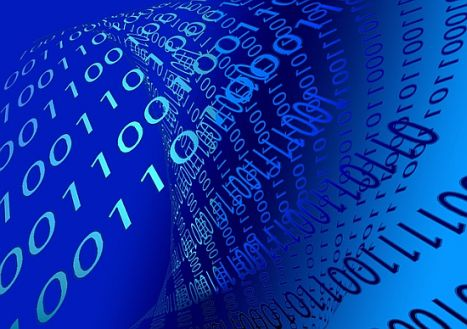# Why do computers only use binary dataThough we see modern day computers do everything tasks as simple as performing a calculation to more complex tasks as controlling a rover on another planet, the secret is that computers only understand two digits that are 0 and 1. These two digits are the “binary data”, which all computers of the modern world understand and make use of. However, now the obvious question that comes into the mind of all computer users is why do digits as simple as 99 not directly fed into the computer and instead a binary code has to be generated by the system itself, which for 99 reads something more complex like 1100011?

The answer is relatively simple – modern day computers are digital and unlike the older “analog” computers, they understand that there are only two states of something – “on” and “off”. And to make them understand these two states, the digit 1 means something is on and the digit 0 means that something is off. This state directly corresponds to there being an electrical current present or absent. Each binary digit, or simply one bit, is a single 0 or 1, which corresponds to the state of a single switch in a circuit. Adding hundreds of even thousands of these switches together can represent more numbers and hence it enables the computer to perform a wide variety of calculations.

Here the common misconception is that binary uses more storage than a decimal base (base 10) in representation of digits. For example, when you read 99, you just have to read and store two digits in your mind that is two 9s, however, the binary representation of the same is 1100011, which adds up to seven digits. However, this is an invalid argument in the context of displaying numbers on the screen, since they have to be stored in binary regardless, the only reason that 99 looks smaller than 1100011 is because of the way we usually write something on paper. Increasing the base from two to 10 would definitely decrease the number of digits required to represent any number, but it won’t work with computers as there cannot be more than two states of a transistor other than “on” and “off”, obviously you get into quantum computers for the same where there can be more than two states.

In simple words, computers only make use of binary because we currently don’t have the technology to create switches that can operate in more than the two possible states. The binary system was chosen for its ease of use inside a computer’s system where transistors can get an electrical current or not.Written by:
Editor-in-Chief and lead author at WhyDo